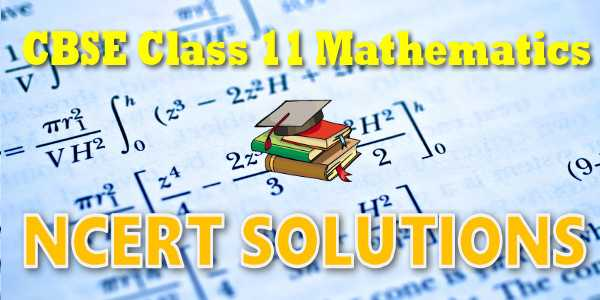# NCERT solutions for class 11 Mathematics## NEET-UG 2021

10,000+ Daily Practice Questions

(only at ₹ 299/- in myCBSEguide App)

## NCERT solutions for class 11 Mathematics## myCBSEguide App

Complete Guide for CBSE Students

NCERT Solutions, NCERT Exemplars, Revison Notes, Free Videos, CBSE Papers, MCQ Tests & more.## NCERT Solutions Chapter 8: BINOMIAL THEORY

In earlier classes, we have learnt how to find the squares and cubes of binomials like a + b and a-b. Using them, we could evaluate the numericals values of number like (98) 2 =(100-2)2,(999) 3 =(1000-1)3etc. However, for higher powers like (101) 5 ., the calculations become difficult by using repeated multiplication.This difficulty was overcome by a theorem known as binomial theorem. It gives an easier way to expand (a+b),where n is an integer or a rational number. In this chapter, we study binomial theorem for positive integral indices only.

## NCERT Solutions for Class 11th Mathematics

NCERT Solutions Class 11 Mathematics PDF (Download) Free from myCBSEguide app and myCBSEguide website. Ncert solution class 11 Mathematics includes text book solutions from book. NCERT Solutions for CBSE Class 11 maths have total 15 chapters. Class 11 Mathematics ncert Solutions in pdf for free Download are given in this website. Ncert Mathematics class 11 solutions PDF and Mathematics ncert class 11 PDF solutions with latest modifications and as per the latest CBSE syllabus are only available in myCBSEguide. NCERT 11 Mathematics Text book Solutions.

## NCERT Solutions for Class 11## myCBSEguide

Trusted by 1 Crore+ Students

#### CBSE Test Generator

Create papers in minutes

Print with your name & Logo

3 Lakhs+ Questions

Solutions Included

Based on CBSE Blueprint

Best fit for Schools & Tutors

#### Work from Home

• Work from home with us
• Create questions or review them from home

No software required, no contract to sign. Simply apply as teacher, take eligibility test and start working with us. Required desktop or laptop with internet connection